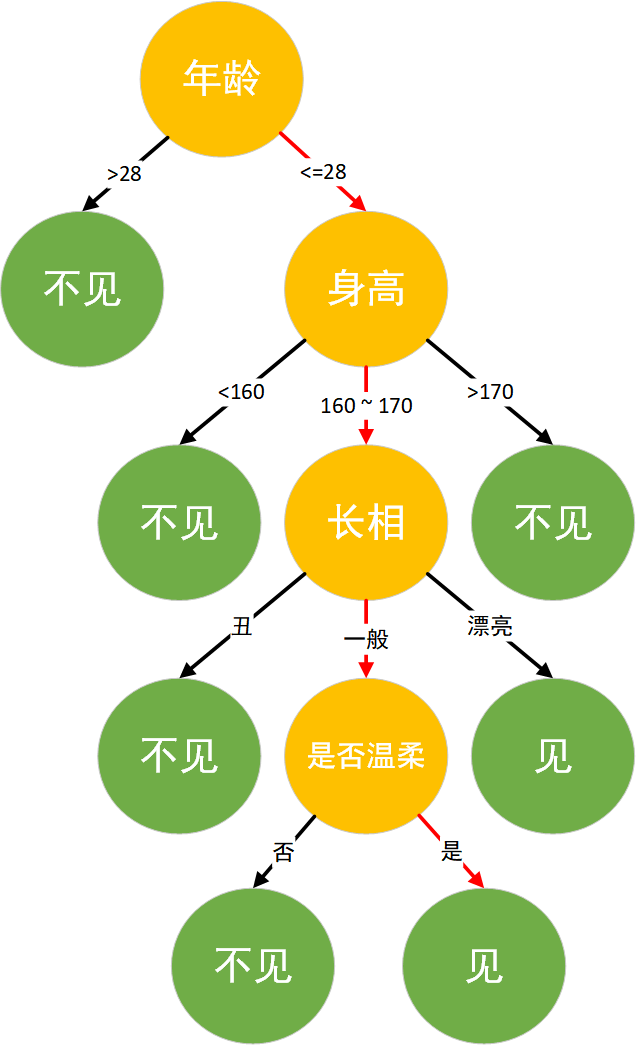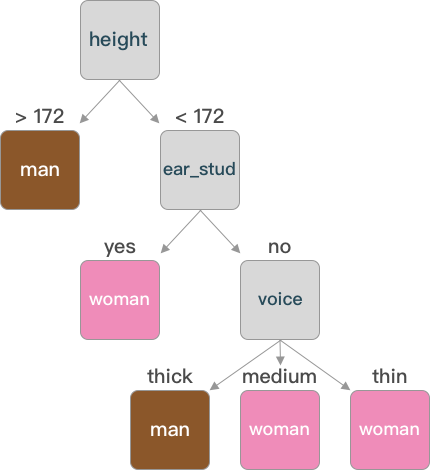# 机器学习|决策树详解## 决策树算法实现

• 第 1 步: 数据准备：通过数据清洗和数据处理，将数据整理为没有缺省值的向量。
• 第 2 步: 寻找最佳特征：遍历每个特征的每一种划分方式，找到最好的划分特征。
• 第 3 步: 生成分支：划分成两个或多个节点。
• 第 4 步: 生成决策树：对分裂后的节点分别继续执行2-3步，直到每个节点只有一种类别。
• 第 5 步: 决策分类：根据训练决策树模型，将预测数据进行分类。

### 数据生成

• hair:头发长短(long:长,short:短)
• voice:声音粗细(thick:粗,thin:细)
• height:身高
• ear_stud:是否带有耳钉(yes:是,no:没有)

|

|

|

|

|

|

### 划分选择

#### 信息增益（ID3）

Ent(D)=−|y|∑k=1pklog2pk(1)

$$(1)Ent(D)=−∑k=1|y|pklog2pk$$

Ent(data)=−2∑k=1pklog2pk=−(817log2817+917log2917)=0.9975

$$Ent(data)=−∑k=12pklog2pk=−(817log2817+917log2917)=0.9975$$

|

|

|

|

|

|

Gain(D,x)=Ent(D)−M∑m=1|Dm||D|Ent(Dm)(2)

$$(2)Gain(D,x)=Ent(D)−∑m=1M|Dm||D|Ent(Dm)$$

Ent(D1)=−(39log239+69log269)=0.449

$$Ent(D1)=−(39log239+69log269)=0.449$$

Ent(D2)=−(58log258+38log238)=0.486

$$Ent(D2)=−(58log258+38log238)=0.486$$

Gain(D,hair)=Ent(D)−2∑m=1|Dm||D|Ent(Dm)=0.9975−(917∗0.449+817∗0.486)=0.062

$$Gain(D,hair)=Ent(D)−∑m=12|Dm||D|Ent(Dm)=0.9975−(917∗0.449+817∗0.486)=0.062$$

|

|

|

|

|

|

#### 信息增益率（C4.5）

GainRatio(D,a)=Gain(D,a)IV(a)(3)

$$(3)GainRatio(D,a)=Gain(D,a)IV(a)$$

IV(a)=−M∑m=1|Dm||D|log2|Dm||D|(4)

$$(4)IV(a)=−∑m=1M|Dm||D|log2|Dm||D|$$

IV(a)$IV(a)$ 称为特征 a$a$ 的固有值，当 a$a$ 的取值数目越多，则 IV(a)$IV(a)$ 的值通常会比较大。例如：

IV(hair)=−917log2917−817log2817=0.998

$$IV(hair)=−917log2917−817log2817=0.998$$

IV(voice)=−717log2717−517log2517−517log2517=1.566

$$IV(voice)=−717log2717−517log2517−517log2517=1.566$$

### 连续值处理

Gain(D,X)=Ent(D)−|D<t||D|Ent(D<t)−|D>t||D|Ent(D>t)(5)

$$(5)Gain(D,X)=Ent(D)−|D<t||D|Ent(D<t)−|D>t||D|Ent(D>t)$$

|

|

|

|

|

|

### 算法实现

|

|

|

|

|

|

|

|

|

#### 构建决策树

|

|

|

|

|

||

|

|

|

|

|

|

|

|

|

|

|

## 预测分类

### 导入数据

|

|

|

• school：学生所读学校(GPMS)
• sex: 性别(F：女，M：男)
• address: 家庭住址(U：城市，R：郊区)
• Pstatus: 父母状态(A：同居，T：分居)
• Pedu: 父母学历由低到高
• reason: 选择这所学校的原因(home：家庭,course：课程设计，reputation：学校地位，other：其他)
• guardian: 监护人(mother：母亲，father：父亲，other：其他)
• studytime: 周末学习时长
• schoolsup: 额外教育支持(yes：有，no：没有)
• famsup: 家庭教育支持(yes：有，no：没有)
• paid: 是否上补习班(yes：是，no：否)
• higher: 是否想受更好的教育(yes：是，no：否)
• internet: 是否家里联网(yes：是，no：否)
• G1: 一阶段测试成绩
• G2: 二阶段测试成绩
• G3: 最终成绩

|

|

|

|

|

|

|

|

|

|

|

|

|

|

|

### 数据划分

|

|

|

• x_train,x_test, y_train, y_test 分别表示，切分后的 特征的训练集，特征的测试集，标签的训练集，标签的测试集；其中特征和标签的值是一一对应的。
• train_data,train_target分别表示为待划分的特征集和待划分的标签集。
• test_size：测试样本所占比例。
• random_state：随机数种子,在需要重复实验时，保证在随机数种子一样时能得到一组一样的随机数。

|

|

|

### 决策树构建

|

|

|

• criterion 表示特征划分方法选择，默认为 gini (在后面会讲到)，可选择为 entropy (信息增益)。
• ramdom_state 表示随机数种子，当特征特别多时 scikit-learn 为了提高效率，随机选取部分特征来进行特征选择，即找到所有特征中较优的特征。

• fit(x,y)训练决策树。
• predict(X) 对数据集进行预测返回预测结果。

|

|

|

### 决策树可视化

|

|

|

|

|

|svg

|

|

|

### 分类准确率计算

accur=∑Ni=1I(¯yi=yi)N(6)

$$(6)accur=∑i=1NI(yi¯=yi)N$$

|

|

|

## CART 决策树

CART决策树的构建和常见的 ID3C4.5 算法的流程相似，但在特征划分选择上CART选择了 基尼指数 作为划分标准。数据集 D$D$ 的纯度可用基尼值来度量：

Gini(D)=|y|∑y=1∑k′≠kpkp′k(7)

$$(7)Gini(D)=∑y=1|y|∑k′≠kpkpk′$$

GiniValue(D,a)=M∑m=1|Dm||D|Gini(Dm)(8)

$$(8)GiniValue(D,a)=∑m=1M|Dm||D|Gini(Dm)$$

laugh12321

2019-02-01

2020-10-23